# RD Sharma Solutions For Class 7 Maths Exercise 8.2 Chapter 8 Linear Equations in One Variable

Students can view and download the PDF of RD Sharma Solutions for Class 7 Maths Exercise 8.2 of Chapter 8 Linear Equations in One Variable are available here. The BYJU’S experts in Maths formulate the questions present in this exercise. This exercise explains about solving linear equations. One of the methods is the systematic method which includes:

• Equation involving subtraction
• Equation involving multiplication
• Equation involving division
• Equation solvable by using more than one rule or operation

By practising RD Sharma Solutions for Class 7, students will able to solve problems with Math skills.

## Download the PDF of RD Sharma Solutions For Class 7 Maths Chapter 8 – Linear Equations in One variable Exercise 8.2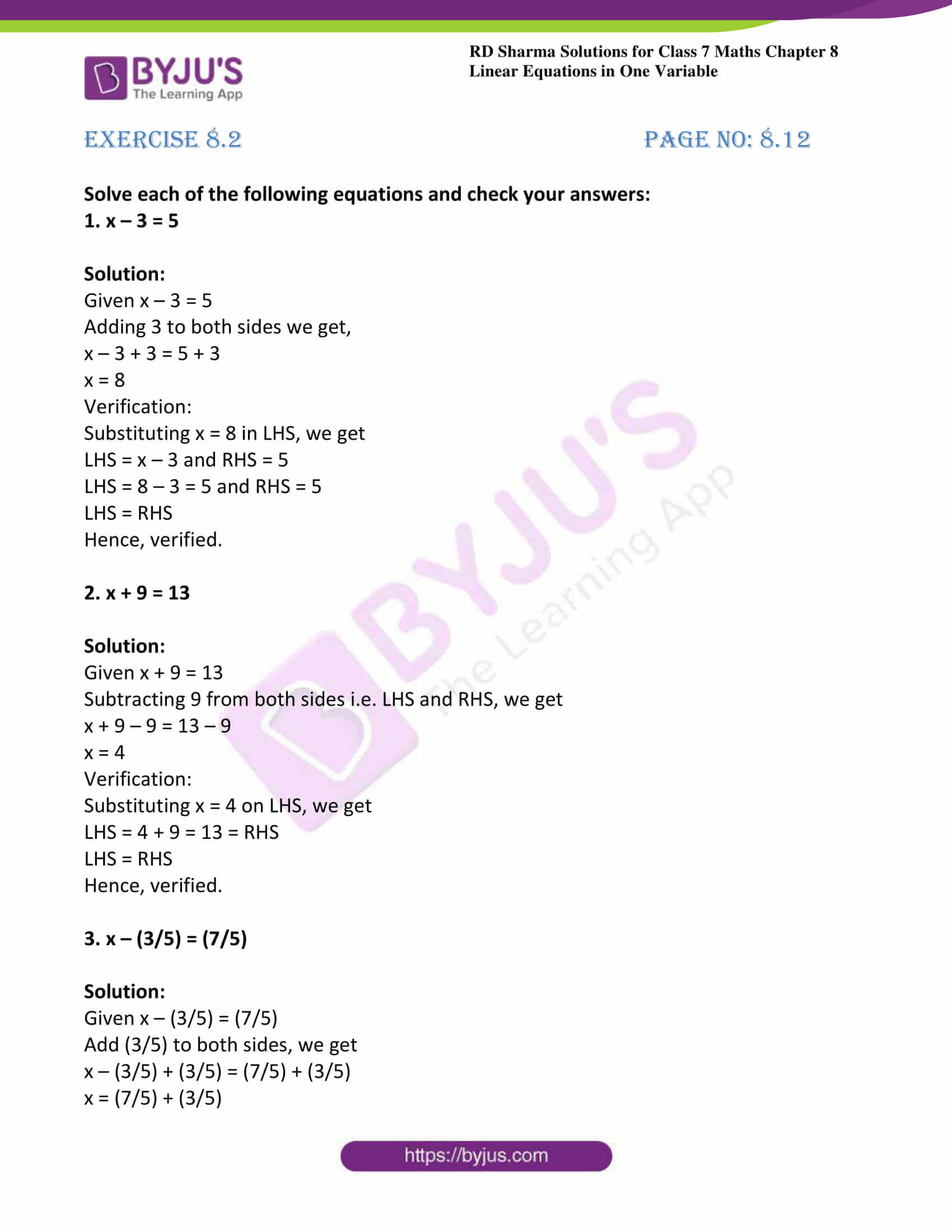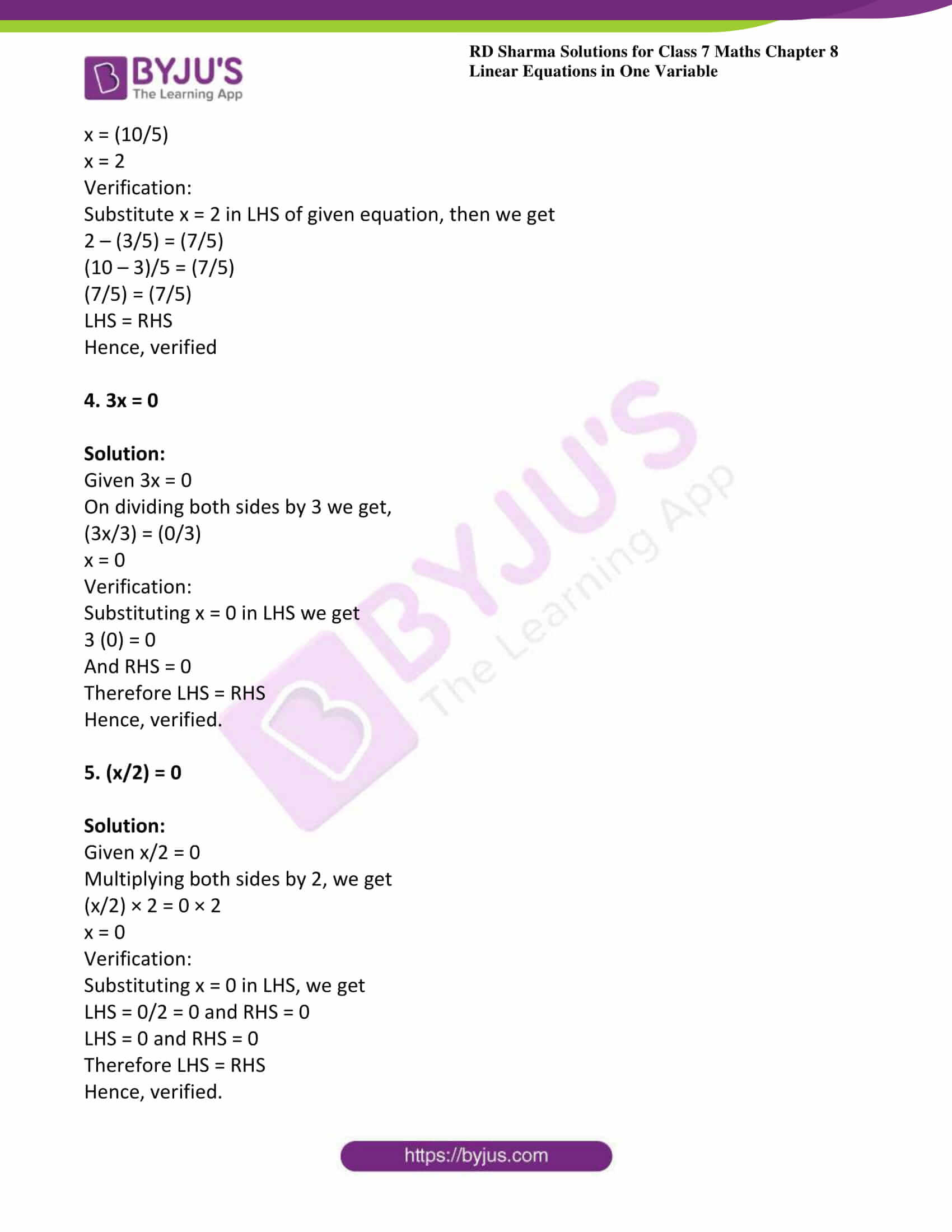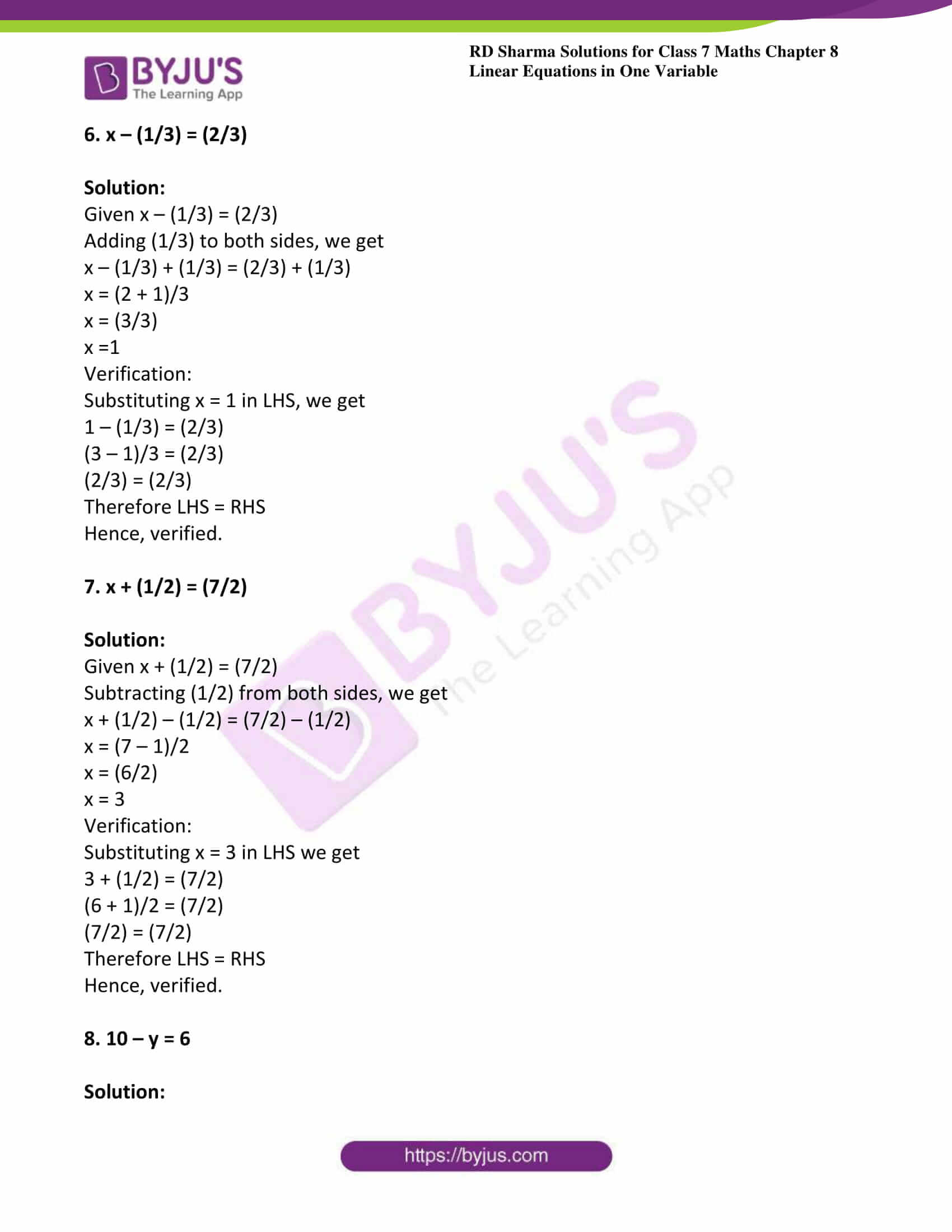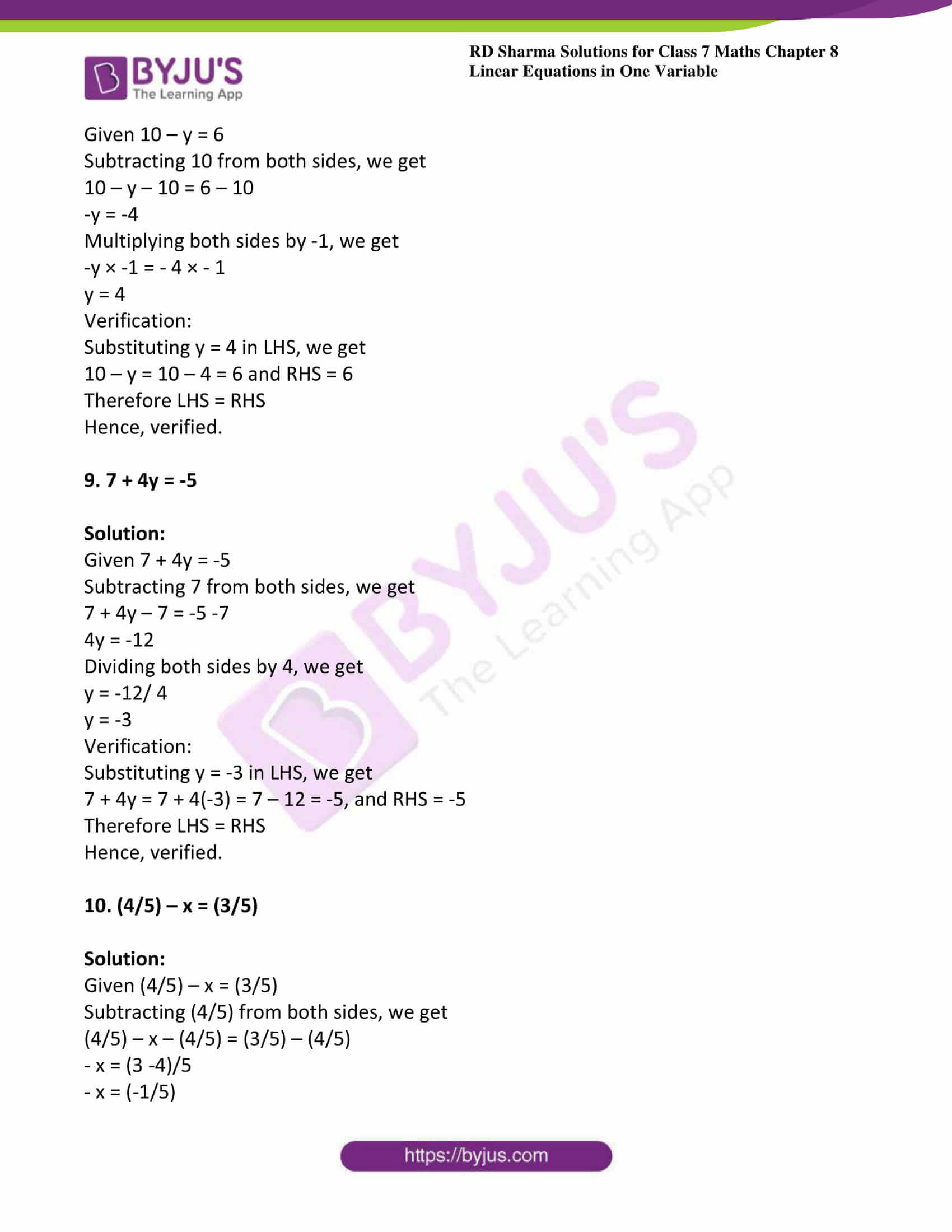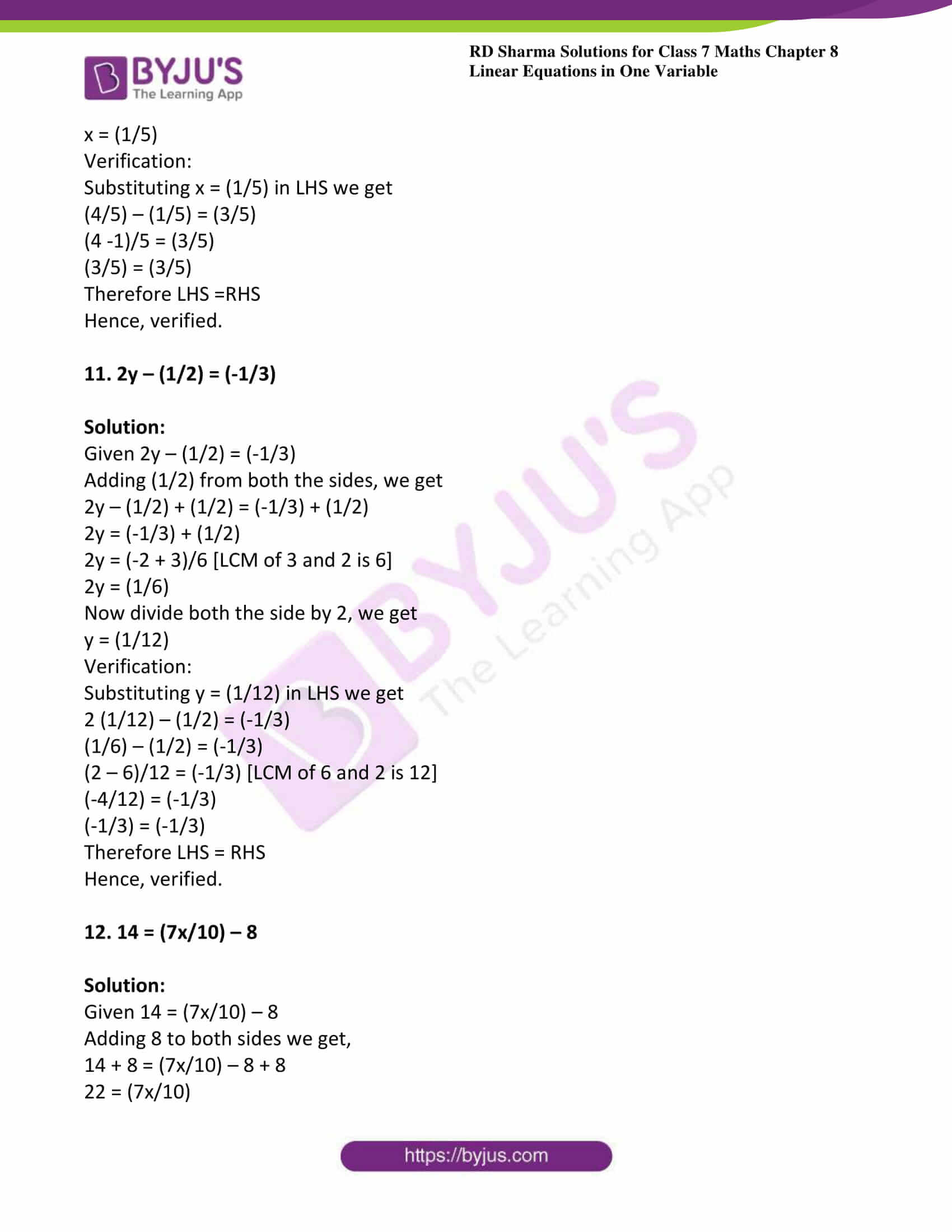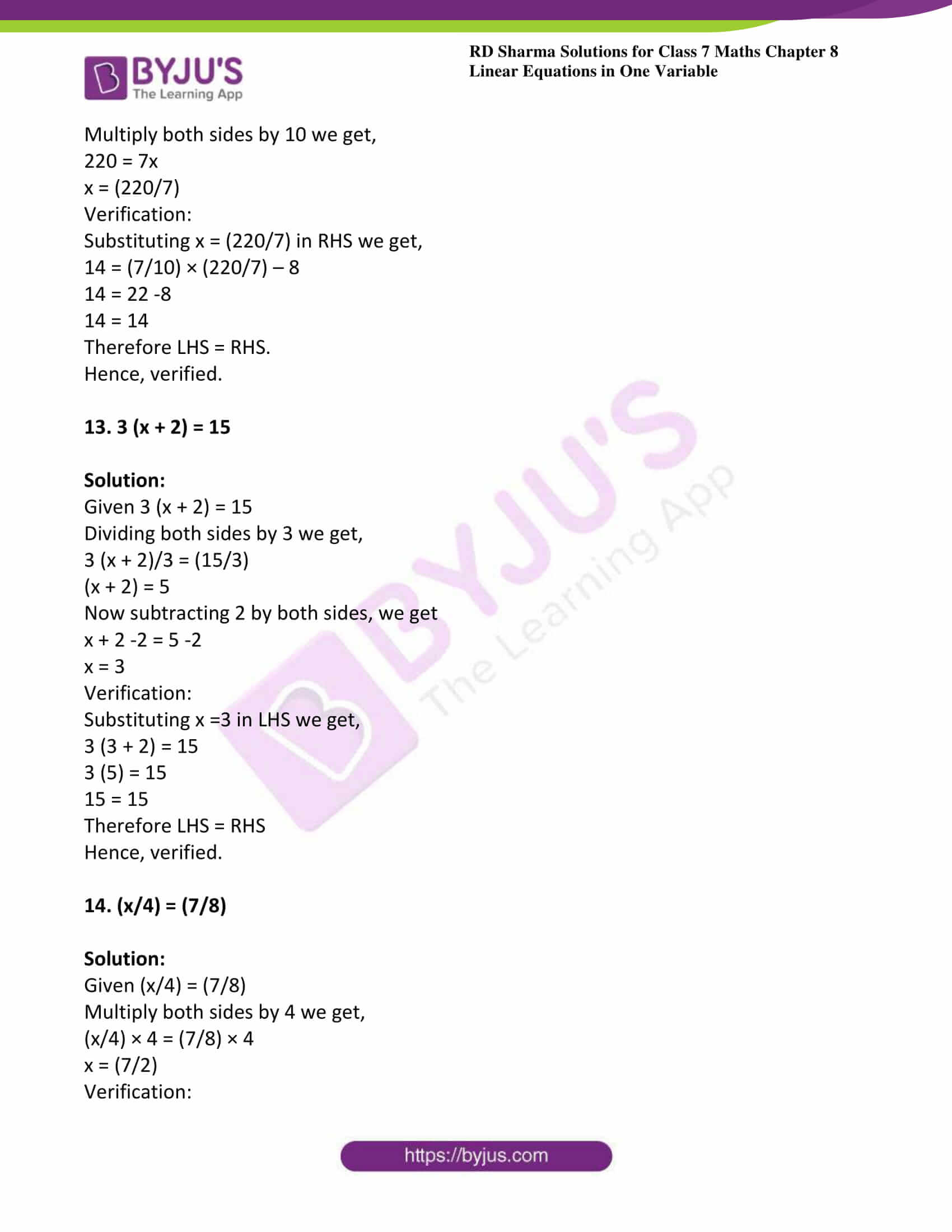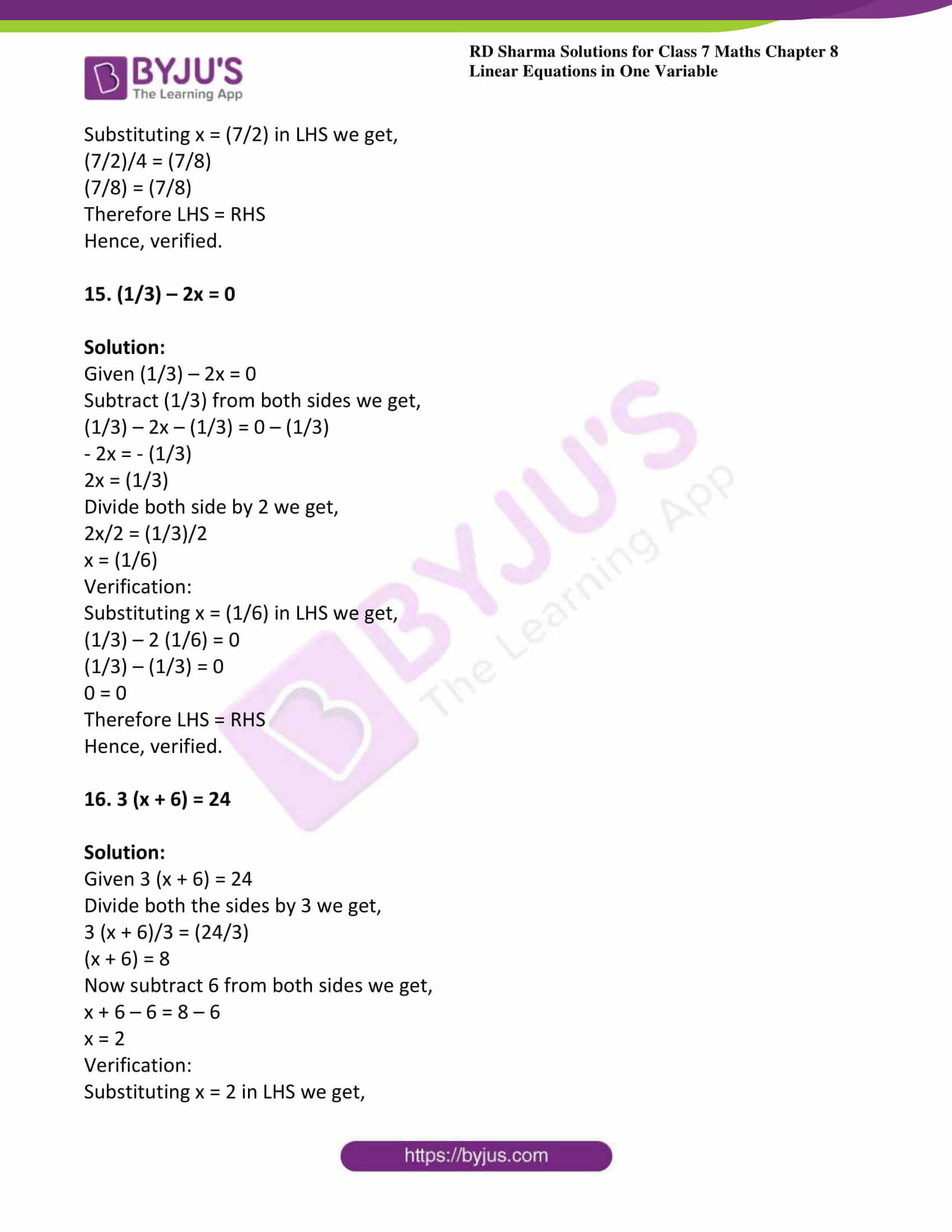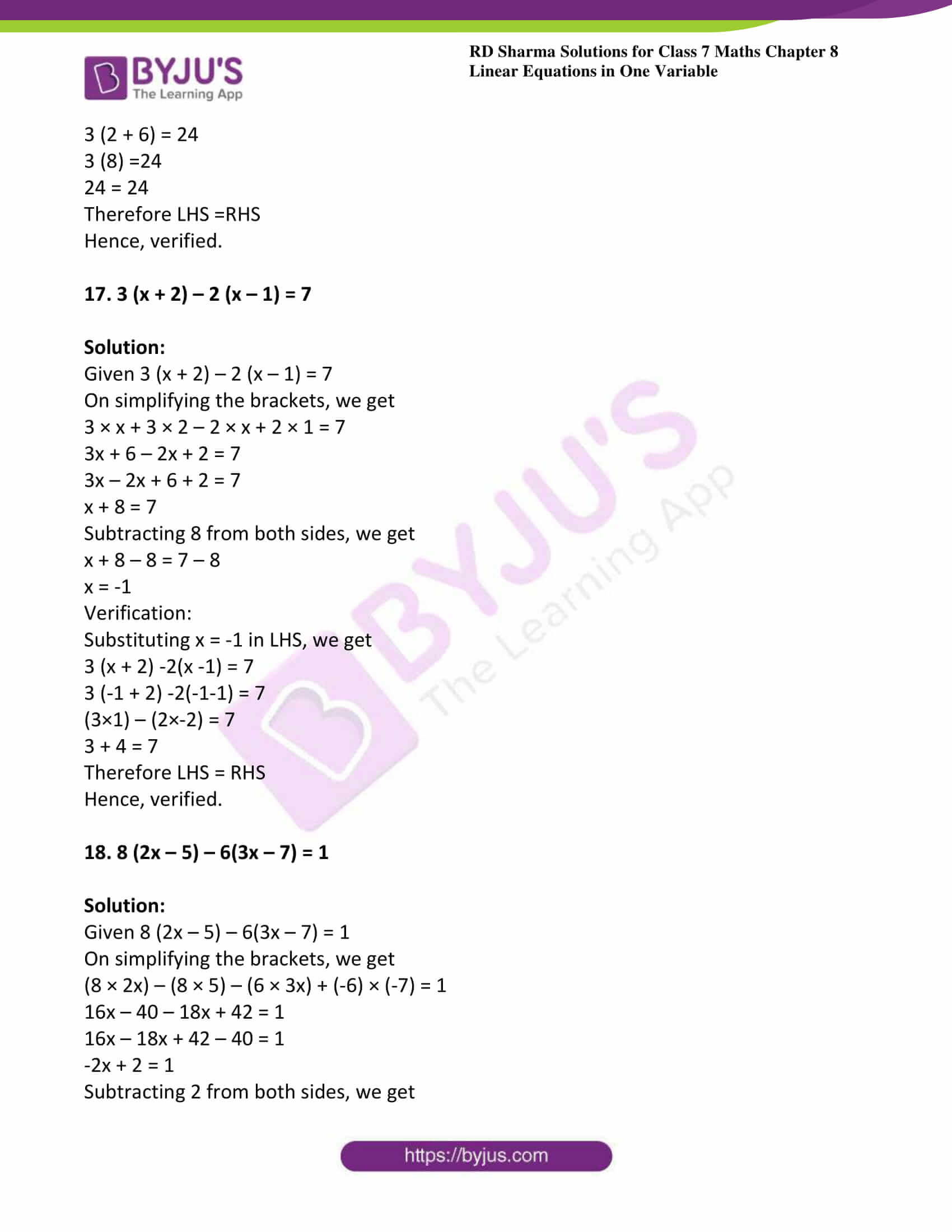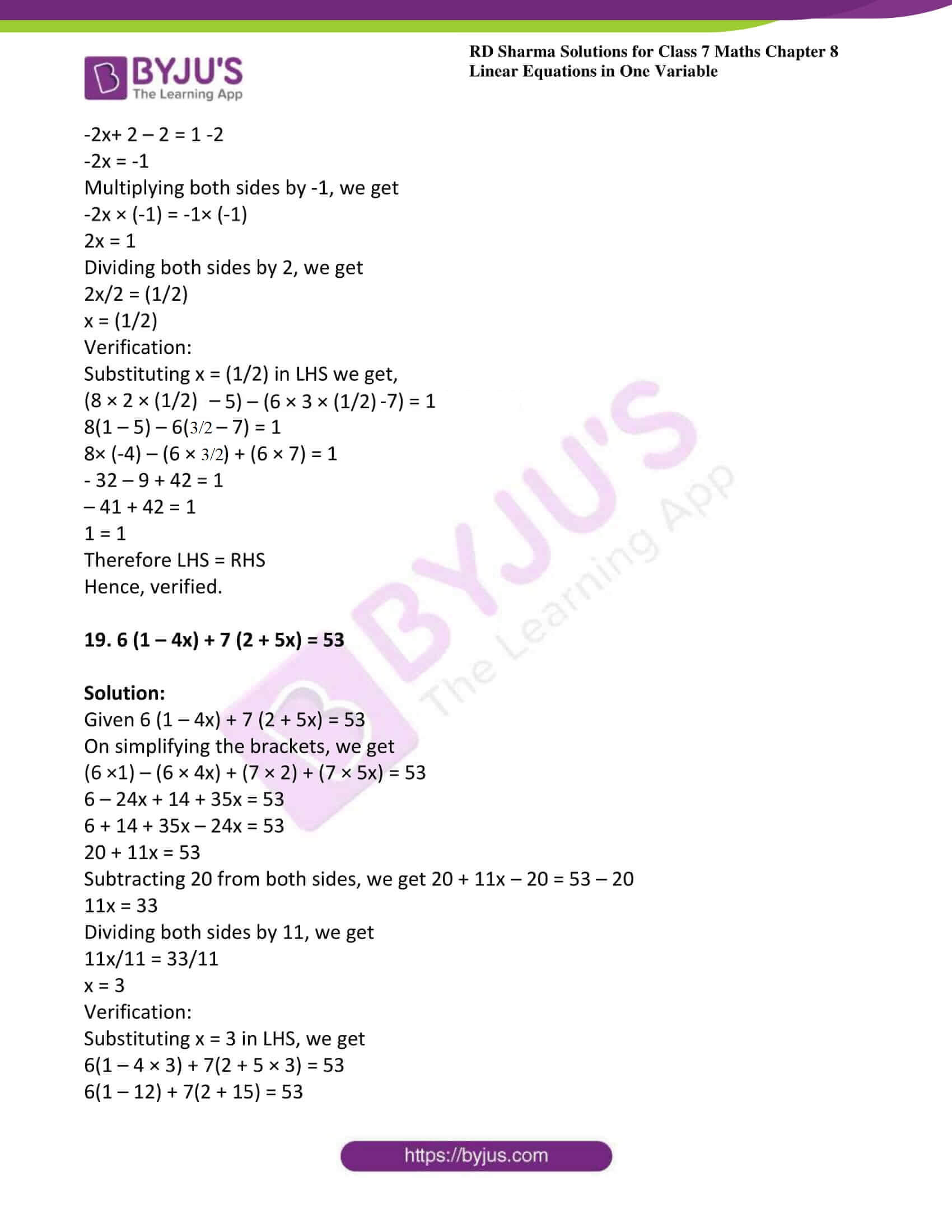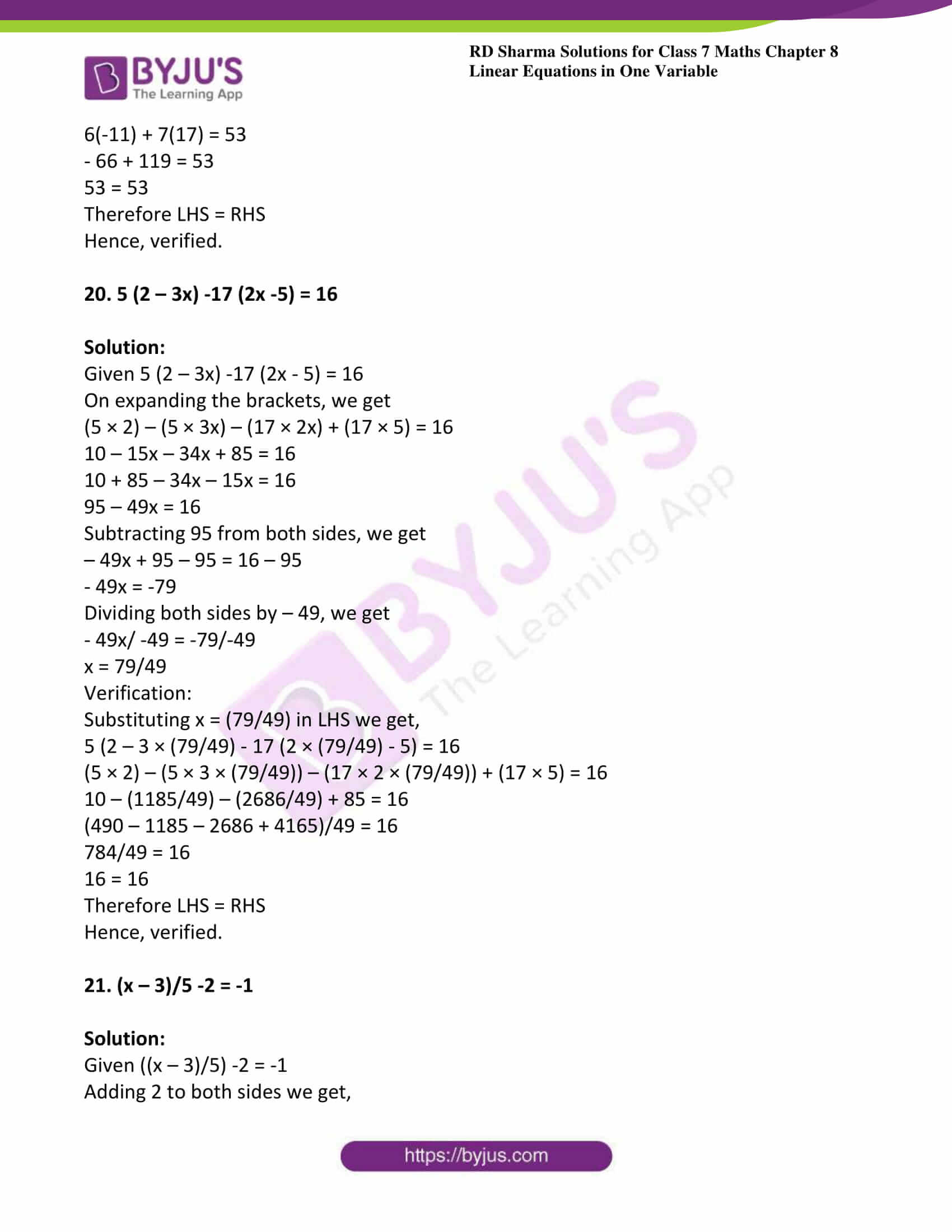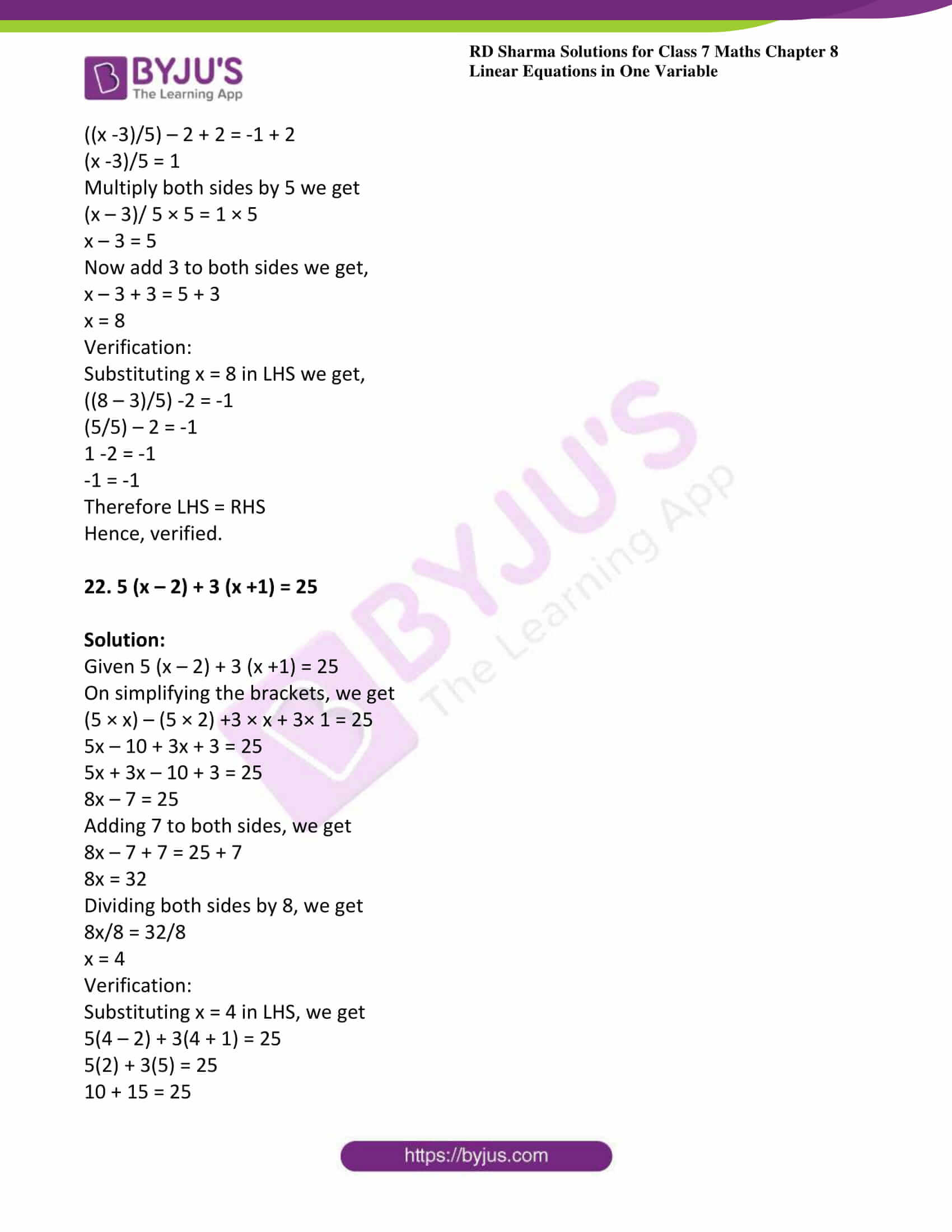### Access answers to Maths RD Sharma Solutions For Class 7 Chapter 8 – Linear Equation in One Variable Exercise 8.2

1. x – 3 = 5

Solution:

Given x – 3 = 5

Adding 3 to both sides we get,

x – 3 + 3 = 5 + 3

x = 8

Verification:

Substituting x = 8 in LHS, we get

LHS = x – 3 and RHS = 5

LHS = 8 – 3 = 5 and RHS = 5

LHS = RHS

Hence, verified.

2. x + 9 = 13

Solution:

Given x + 9 = 13

Subtracting 9 from both sides i.e. LHS and RHS, we get

x + 9 – 9 = 13 – 9

x = 4

Verification:

Substituting x = 4 on LHS, we get

LHS = 4 + 9 = 13 = RHS

LHS = RHS

Hence, verified.

3. x – (3/5) = (7/5)

Solution:

Given x – (3/5) = (7/5)

Add (3/5) to both sides, we get

x – (3/5) + (3/5) = (7/5) + (3/5)

x = (7/5) + (3/5)

x = (10/5)

x = 2

Verification:

Substitute x = 2 in LHS of given equation, then we get

2 – (3/5) = (7/5)

(10 – 3)/5 = (7/5)

(7/5) = (7/5)

LHS = RHS

Hence, verified

4. 3x = 0

Solution:

Given 3x = 0

On dividing both sides by 3 we get,

(3x/3) = (0/3)

x = 0

Verification:

Substituting x = 0 in LHS we get

3 (0) = 0

And RHS = 0

Therefore LHS = RHS

Hence, verified.

5. (x/2) = 0

Solution:

Given x/2 = 0

Multiplying both sides by 2, we get

(x/2) × 2 = 0 × 2

x = 0

Verification:

Substituting x = 0 in LHS, we get

LHS = 0/2 = 0 and RHS = 0

LHS = 0 and RHS = 0

Therefore LHS = RHS

Hence, verified.

6. x – (1/3) = (2/3)

Solution:

Given x – (1/3) = (2/3)

Adding (1/3) to both sides, we get

x – (1/3) + (1/3) = (2/3) + (1/3)

x = (2 + 1)/3

x = (3/3)

x =1

Verification:

Substituting x = 1 in LHS, we get

1 – (1/3) = (2/3)

(3 – 1)/3 = (2/3)

(2/3) = (2/3)

Therefore LHS = RHS

Hence, verified.

7. x + (1/2) = (7/2)

Solution:

Given x + (1/2) = (7/2)

Subtracting (1/2) from both sides, we get

x + (1/2) – (1/2) = (7/2) – (1/2)

x = (7 – 1)/2

x = (6/2)

x = 3

Verification:

Substituting x = 3 in LHS we get

3 + (1/2) = (7/2)

(6 + 1)/2 = (7/2)

(7/2) = (7/2)

Therefore LHS = RHS

Hence, verified.

8. 10 – y = 6

Solution:

Given 10 – y = 6

Subtracting 10 from both sides, we get

10 – y – 10 = 6 – 10

-y = -4

Multiplying both sides by -1, we get

-y × -1 = – 4 × – 1

y = 4

Verification:

Substituting y = 4 in LHS, we get

10 – y = 10 – 4 = 6 and RHS = 6

Therefore LHS = RHS

Hence, verified.

9. 7 + 4y = -5

Solution:

Given 7 + 4y = -5

Subtracting 7 from both sides, we get

7 + 4y – 7 = -5 -7

4y = -12

Dividing both sides by 4, we get

y = -12/ 4

y = -3

Verification:

Substituting y = -3 in LHS, we get

7 + 4y = 7 + 4(-3) = 7 – 12 = -5, and RHS = -5

Therefore LHS = RHS

Hence, verified.

10. (4/5) – x = (3/5)

Solution:

Given (4/5) – x = (3/5)

Subtracting (4/5) from both sides, we get

(4/5) – x – (4/5) = (3/5) – (4/5)

– x = (3 -4)/5

– x = (-1/5)

x = (1/5)

Verification:

Substituting x = (1/5) in LHS we get

(4/5) – (1/5) = (3/5)

(4 -1)/5 = (3/5)

(3/5) = (3/5)

Therefore LHS =RHS

Hence, verified.

11. 2y – (1/2) = (-1/3)

Solution:

Given 2y – (1/2) = (-1/3)

Adding (1/2) from both the sides, we get

2y – (1/2) + (1/2) = (-1/3) + (1/2)

2y = (-1/3) + (1/2)

2y = (-2 + 3)/6 [LCM of 3 and 2 is 6]

2y = (1/6)

Now divide both the side by 2, we get

y = (1/12)

Verification:

Substituting y = (1/12) in LHS we get

2 (1/12) – (1/2) = (-1/3)

(1/6) – (1/2) = (-1/3)

(2 – 6)/12 = (-1/3) [LCM of 6 and 2 is 12]

(-4/12) = (-1/3)

(-1/3) = (-1/3)

Therefore LHS = RHS

Hence, verified.

12. 14 = (7x/10) – 8

Solution:

Given 14 = (7x/10) – 8

Adding 8 to both sides we get,

14 + 8 = (7x/10) – 8 + 8

22 = (7x/10)

Multiply both sides by 10 we get,

220 = 7x

x = (220/7)

Verification:

Substituting x = (220/7) in RHS we get,

14 = (7/10) × (220/7) – 8

14 = 22 -8

14 = 14

Therefore LHS = RHS.

Hence, verified.

13. 3 (x + 2) = 15

Solution:

Given 3 (x + 2) = 15

Dividing both sides by 3 we get,

3 (x + 2)/3 = (15/3)

(x + 2) = 5

Now subtracting 2 by both sides, we get

x + 2 -2 = 5 -2

x = 3

Verification:

Substituting x =3 in LHS we get,

3 (3 + 2) = 15

3 (5) = 15

15 = 15

Therefore LHS = RHS

Hence, verified.

14. (x/4) = (7/8)

Solution:

Given (x/4) = (7/8)

Multiply both sides by 4 we get,

(x/4) × 4 = (7/8) × 4

x = (7/2)

Verification:

Substituting x = (7/2) in LHS we get,

(7/2)/4 = (7/8)

(7/8) = (7/8)

Therefore LHS = RHS

Hence, verified.

15. (1/3) – 2x = 0

Solution:

Given (1/3) – 2x = 0

Subtract (1/3) from both sides we get,

(1/3) – 2x – (1/3) = 0 – (1/3)

– 2x = – (1/3)

2x = (1/3)

Divide both side by 2 we get,

2x/2 = (1/3)/2

x = (1/6)

Verification:

Substituting x = (1/6) in LHS we get,

(1/3) – 2 (1/6) = 0

(1/3) – (1/3) = 0

0 = 0

Therefore LHS = RHS

Hence, verified.

16. 3 (x + 6) = 24

Solution:

Given 3 (x + 6) = 24

Divide both the sides by 3 we get,

3 (x + 6)/3 = (24/3)

(x + 6) = 8

Now subtract 6 from both sides we get,

x + 6 – 6 = 8 – 6

x = 2

Verification:

Substituting x = 2 in LHS we get,

3 (2 + 6) = 24

3 (8) =24

24 = 24

Therefore LHS =RHS

Hence, verified.

17. 3 (x + 2) – 2 (x – 1) = 7

Solution:

Given 3 (x + 2) – 2 (x – 1) = 7

On simplifying the brackets, we get

3 × x + 3 × 2 – 2 × x + 2 × 1 = 7

3x + 6 – 2x + 2 = 7

3x – 2x + 6 + 2 = 7

x + 8 = 7

Subtracting 8 from both sides, we get

x + 8 – 8 = 7 – 8

x = -1

Verification:

Substituting x = -1 in LHS, we get

3 (x + 2) -2(x -1) = 7

3 (-1 + 2) -2(-1-1) = 7

(3×1) – (2×-2) = 7

3 + 4 = 7

Therefore LHS = RHS

Hence, verified.

18. 8 (2x – 5) – 6(3x – 7) = 1

Solution:

Given 8 (2x – 5) – 6(3x – 7) = 1

On simplifying the brackets, we get

(8 × 2x) – (8 × 5) – (6 × 3x) + (-6) × (-7) = 1

16x – 40 – 18x + 42 = 1

16x – 18x + 42 – 40 = 1

-2x + 2 = 1

Subtracting 2 from both sides, we get

-2x+ 2 – 2 = 1 -2

-2x = -1

Multiplying both sides by -1, we get

-2x × (-1) = -1× (-1)

2x = 1

Dividing both sides by 2, we get

2x/2 = (1/2)

x = (1/2)

Verification:

Substituting x = (1/2) in LHS we get,

(8 × (2 × (1/2)– 5) – (6 × (3 × (1/2) -7) = 1

8(1 – 5) – 6(3/2 – 7) = 1

8× (-4) – (6 × 3/2) + (6 × 7) = 1

– 32 – 9 + 42 = 1

– 41 + 42 = 1

1 = 1

Therefore LHS = RHS

Hence, verified.

19. 6 (1 – 4x) + 7 (2 + 5x) = 53

Solution:

Given 6 (1 – 4x) + 7 (2 + 5x) = 53

On simplifying the brackets, we get

(6 ×1) – (6 × 4x) + (7 × 2) + (7 × 5x) = 53

6 – 24x + 14 + 35x = 53

6 + 14 + 35x – 24x = 53

20 + 11x = 53

Subtracting 20 from both sides, we get 20 + 11x – 20 = 53 – 20

11x = 33

Dividing both sides by 11, we get

11x/11 = 33/11

x = 3

Verification:

Substituting x = 3 in LHS, we get

6(1 – 4 × 3) + 7(2 + 5 × 3) = 53

6(1 – 12) + 7(2 + 15) = 53

6(-11) + 7(17) = 53

– 66 + 119 = 53

53 = 53

Therefore LHS = RHS

Hence, verified.

20. 5 (2 – 3x) -17 (2x -5) = 16

Solution:

Given 5 (2 – 3x) -17 (2x – 5) = 16

On expanding the brackets, we get

(5 × 2) – (5 × 3x) – (17 × 2x) + (17 × 5) = 16

10 – 15x – 34x + 85 = 16

10 + 85 – 34x – 15x = 16

95 – 49x = 16

Subtracting 95 from both sides, we get

– 49x + 95 – 95 = 16 – 95

– 49x = -79

Dividing both sides by – 49, we get

– 49x/ -49 = -79/-49

x = 79/49

Verification:

Substituting x = (79/49) in LHS we get,

5 (2 – 3 × (79/49) – 17 (2 × (79/49) – 5) = 16

(5 × 2) – (5 × 3 × (79/49)) – (17 × 2 × (79/49)) + (17 × 5) = 16

10 – (1185/49) – (2686/49) + 85 = 16

(490 – 1185 – 2686 + 4165)/49 = 16

784/49 = 16

16 = 16

Therefore LHS = RHS

Hence, verified.

21. (x – 3)/5 -2 = -1

Solution:

Given ((x – 3)/5) -2 = -1

Adding 2 to both sides we get,

((x -3)/5) – 2 + 2 = -1 + 2

(x -3)/5 = 1

Multiply both sides by 5 we get

(x – 3)/ 5 × 5 = 1 × 5

x – 3 = 5

Now add 3 to both sides we get,

x – 3 + 3 = 5 + 3

x = 8

Verification:

Substituting x = 8 in LHS we get,

((8 – 3)/5) -2 = -1

(5/5) – 2 = -1

1 -2 = -1

-1 = -1

Therefore LHS = RHS

Hence, verified.

22. 5 (x – 2) + 3 (x +1) = 25

Solution:

Given 5 (x – 2) + 3 (x +1) = 25

On simplifying the brackets, we get

(5 × x) – (5 × 2) +3 × x + 3× 1 = 25

5x – 10 + 3x + 3 = 25

5x + 3x – 10 + 3 = 25

8x – 7 = 25

Adding 7 to both sides, we get

8x – 7 + 7 = 25 + 7

8x = 32

Dividing both sides by 8, we get

8x/8 = 32/8

x = 4

Verification:

Substituting x = 4 in LHS, we get

5(4 – 2) + 3(4 + 1) = 25

5(2) + 3(5) = 25

10 + 15 = 25

25 = 25

Therefore LHS = RHS

Hence, verified.